### Home > GC > Chapter 7 > Lesson 7.2.3 > Problem7-70

7-70.
1. For each diagram below, solve for x. Show all work. Homework Help ✎

1.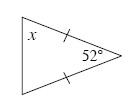2.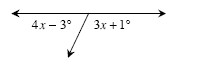3.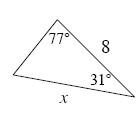4.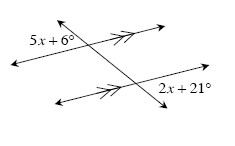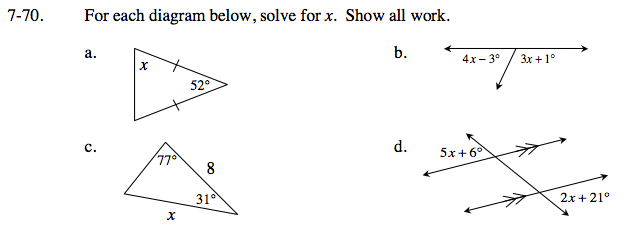If the sides are equal, what does that mean about the angle measures?

x = 64°

What is the measure of a straight angle?